# Overview of the Package trinROC

#### 2021-06-29

The package trinROC helps to assess three-class Receiver Operating Characteristic (ROC) type data. It provides functions for three statistical tests as described in Noll et al. (2019) along with functions for Exploratory Data Analysis (EDA) and visualization.

We assume that the reader has some background in ROC analysis as well as some understanding of the terminology associated with Area Under the ROC Curve (AUC) and Volume Under the ROC Surface (VUS) indices. See Nakas (2014) or Noll et al. (2019) for a concise overview.

This vignette consists of the following parts:

• Short background of the tests
• Testing and comparing markers
• Calculating empirical power curves
• Additional functionality of the package

The package also contains two small data sets cancer and krebs mimicking results of clinical studies. The datasets are fairly small and used in this vignette (and in the examples of the help files) to illustrate the functionality of the functions.

# Short background of the tests

We assume a three-class setting, where one or more classifier yields measurements $$X = x$$ on a continuous scale for the groups of healthy ($$D^-$$), intermediate ($$D^0$$) and diseased ($$D^+$$) individuals. By convention, larger values of $$x$$ represent a more severe status of the disease. The package trinROC provides statistical tests to assess the discriminatory power of such classifiers. These tests are:

• Trinormal based ROC test (trinROC.test), developed by Noll et al. (2019);
• Trinormal VUS test (trinVUS.test), developed by Xiong et al. (2007);
• Bootstrap test, (boot.test), developed by Nakas and Yiannoutsos (2004).

In this document, we refer to the tests by the corresponding R function name. All tests can assess a single classifier, as well as compare two paired or unpaired classifiers.

As their names suggest, the underlying testing approach is different and either based on VUS or on ROC. Hence, their null hypotheses differ as well, as illustrated now.

## VUS based statistical tests

Given two classifiers, boot.test and trinVUS.test are based on the null hypothesis $$VUS_1 = VUS_2$$ with the $$Z$$-statistic

\begin{align*} Z = \frac{\widehat{VUS}_1 - \widehat{VUS}_2}{\sqrt{\widehat{Var}(\widehat{VUS}_1) + \widehat{Var}(\widehat{VUS}_2) - 2 \widehat{Cov}(\widehat{VUS}_1,\widehat{VUS}_2)}}. \end{align*}

If the data of the two classifiers is unpaired, the term $$Cov(VUS_1,VUS_2)$$ is zero. If a single classifier is investigated, the null hypothesis is $$VUS_1 = 1/6$$ with the $$Z$$-statistic

\begin{align*} Z = \frac{\widehat{VUS}_1 - 1/6}{\sqrt{\widehat{Var}(\widehat{VUS}_1)}}, \end{align*}

which is equivalent to compared the VUS of the classifier to the volume of an uninformative classifier. Details about the estimators are given in the aforementioned papers.

## The trinormal based ROC test

In the trinormal model, the ROC surface is given by

\begin{align*} ROC(t_-,t_+) = \Phi \left(\frac{\Phi^{-1} (1-t_+) + d}{ c} \right) - \Phi \left(\frac{\Phi^{-1} (t_-)+b}{a} \right), \end{align*}

where $$\Phi$$ is the cdf of the standard normal distribution and $$a$$, $$b$$, $$c$$ and $$d$$ are functions of the means and standard deviations of the three groups:

\begin{align*} a = \frac{{\sigma}_0}{{\sigma}_-}, \qquad b = \frac{ {\mu}_- - {\mu}_0}{{\sigma}_-}, \qquad c = \frac{{\sigma}_0}{{\sigma}_+}, \qquad d = \frac{ {\mu}_+ - {\mu}_0}{{\sigma}_+}. \end{align*}

Given two classifiers, trinROC.test investigates the shape of the two ROC surfaces. Hence, the resulting null hypothesis is $$a_1=a_2$$, $$b_1=b_2$$, $$c_1=c_2$$ and $$d_1=d_2$$, i.e., the the surfaces have the same shape. Under the null hypothesis, the test statistic

\begin{align*} \chi^2 = \begin{pmatrix} \widehat{a}_1 - \widehat{a}_2 &\widehat{b}_1 -\widehat{b}_2 & \widehat{c}_1-\widehat{c}_2 & \widehat{d}_1-\widehat{d}_2 \end{pmatrix} { \widehat{\boldsymbol{W}}}^{-1} \begin{pmatrix} \widehat{a}_1 - \widehat{a}_2 \\ \widehat{b}_1 - \widehat{b}_2 \\ \widehat{c}_1-\widehat{c}_2 \\ \widehat{d}_1- \widehat{d}_2 \end{pmatrix}, \end{align*}

is distributed approximately as a chi-squared random variables with four degrees of freedom, where $$\widehat{\boldsymbol{W}}$$ contains the corresponding estimated variances and covariances. The test rejects if $$\chi^2 > \chi_{\alpha}^2$$, i.e., if the test statistics exceeds the chi-squared quantile with four degrees of freedom of a pre-defined confidence level $$\alpha$$.

When the data is unpaired, $$\widehat{a}_1$$, $$\widehat{b}_1$$, $$\widehat{c}_1$$, $$\widehat{d}_1$$ are independent from $$\widehat{a}_2$$, $$\widehat{b}_2$$, $$\widehat{c}_2$$, $$\widehat{d}_2$$, respectively, and hence every combination of covariances between them is zero.

It is also possible to investigate a single classifier with the above method. Instead of an existing second classifier, we compare the estimates $$\widehat{a}_1$$ , $$\widehat{b}_1$$, $$\widehat{c}_1$$ and $$\widehat{d}_1$$ of a single marker with those from an artificial marker. If we want to detect whether a single marker is significantly better in allocating individuals to the three classes than a random allocation function we would set the parameters of our null hypothesis $$a_{Ho} = 1= c_{Ho}$$, as we assume equal spread in the classes and $$b_{Ho} = 0 = d_{Ho}$$, as we impose equal means. This yields the null hypothesis $$a_1=1$$, $$b_1=0$$, $$c_1=1$$, $$d_1=0$$, which leads to the chi-squared test

\begin{align*} {\chi}^2 = & \begin{pmatrix} \widehat{a}_1 -1 & \widehat{b}_1 & \widehat{c}_1 -1 & \widehat{d}_1 \end{pmatrix} \widehat{\boldsymbol{W}}^{-1} \begin{pmatrix} \widehat{a}_1 -1 \\ \widehat{b}_1 \\ \widehat{c}_1 - 1 \\ \widehat{d}_1 \end{pmatrix}. \end{align*}

# Testing and comparing markers

We now illustrate the use of the test functions with the artificial dataset cancer.

data(cancer)
str(cancer)
#> 'data.frame':    100 obs. of  10 variables:
#>  $trueClass: Factor w/ 3 levels "healthy","intermediate",..: 3 3 3 3 3 3 3 3 3 3 ... #>$ Class1   : num  1.42 1.69 1.08 1.79 1.46 0.1 1.91 2.57 1 1.87 ...
#>  $Class2 : num 2.31 2.36 1.72 2.34 1.97 0.74 2.82 2.71 1.67 2.51 ... #>$ Class3   : num  -4647 -3694 -5119 -6352 -11509 ...
#>  $Class4 : num -1622958 -1591840 -2299714 -2050907 -2629864 ... #>$ Class5   : num  -476 -473 -618 -564 -901 ...
#>  $Class6 : num 12.36 8.81 9.15 5.52 11.85 ... #>$ Class7   : num  2.48 4.38 2.3 2.41 4.68 1.34 3.18 2.35 1.83 2.83 ...
#>  $Class8 : num 3.02 2.98 2.94 3.01 2.93 2.95 3.02 3.03 2.93 3.06 ... #>$ Class9   : num  -0.75 -0.86 -1.31 -0.27 -1.71 -1.39 -0.46 -1.06 -2.19 -0.91 ...

The first column is a factor indicating the (true) class membership of each individual. The three levels have to be ordered according to heaviness of disease, i.e., healthy, intermediate and diseased (nor the names nor the sorting of the elements plays a role). The other columns contain the measurements yielded by Classifier 1 to 9. Further we note that some columns where multiplied by $$-1$$ in order to fulfill the convention that more diseased individuals have (in general) higher measurements.

## Single marker assessment

For illustration, let us assess Class2 of the data set cancer using the function trinROC.test.

out <- trinROC.test(dat = cancer[,c("trueClass","Class2")])
out
#>
#>  Trinormal based ROC test for single classifier assessment
#>
#> data:  healthy  intermediate  diseased  of  Class2
#> Chi-Squared test = 47.1, df = 4, p-value = 1.4e-09
#> alternative hypothesis: true a1-a2, b1-b1, c1-c2 and d1-d2 is not equal to 0
#> sample estimates:
#>            VUS      a       b       c       d
#> Class2 0.41582 1.8371 -1.2333 0.84364 0.56005

The function returns a list of class "htest", similar to other tests from the stats package. Additionally to the standard list elements, we also obtain detailed information about data and the sample estimates (VUS, and if applicable, estimates of $$a$$, $$b$$, $$c$$ and $$d$$)

out[ c("estimate", "Summary", "CovMat")]
#> $estimate #> VUS a b c d #> Class2 0.41582 1.8371 -1.2333 0.84364 0.56005 #> #>$Summary
#>               n     mu      sd
#> healthy      38 1.3603 0.23525
#> intermediate 25 1.6504 0.43217
#> diseased     37 1.9373 0.51227
#>
#> $CovMat #> [,1] [,2] [,3] [,4] #> [1,] 0.111904 -0.029812 0.0309968 0.0000000 #> [2,] -0.029812 0.181325 0.0000000 0.0619936 #> [3,] 0.030997 0.000000 0.0238526 0.0063849 #> [4,] 0.000000 0.061994 0.0063849 0.0597349 More specifically: • $Summary displays a summary table of $$n_\ell$$, $$\mu_\ell$$ and $$\sigma_\ell$$ for $$\ell = -,0,+$$, the three classes $$D^-$$, $$D^0$$ and $$D^+$$.
• $CovMat or $Sigma displays the covariance matrix of the test.

The tests trinVUS.test and boot.test work analogously.

ROCsin <- trinROC.test(dat = cancer[,c(1,3)])
VUSsin <- trinVUS.test(dat = cancer[,c(1,3)])
bootsin <- boot.test(dat = cancer[,c(1,3)], n.boot = 250)

#>  0.03448
ROCcom$p.value #>  0.13736 # is equal to: x2 <- with(cancer, cancer[trueClass=="healthy", 5]) y2 <- with(cancer, cancer[trueClass=="intermediate", 5]) z2 <- with(cancer, cancer[trueClass=="diseased", 5]) ROCcomp2 <- trinROC.test(x1, y1, z1, x2, y2, z2, paired = TRUE) Beside the argument paired it is also possible to adjust the confidence level (default is conf.level = 0.95). For the trinVUS.test and the boot.test one can also specify the alternative hypothesis (alternative = c("two.sided", "less", "greater")). This not possible for the trinROC.test, as this is a chi-squared test. # Calcuating empirical power curves In this section, we outline the code used to construct the empirical power curves of Figures 2, 3 etc. For simplicity, here and in the paper, we set the mean and variances if the healthy group to zero and one, respectively. The variances of the intermediate and diseased groups are pre-specified (“slight crossing”). Finally the remaining means are found based on a desired VUS. require( ggplot2, quietly = TRUE) require( MASS, quietly = TRUE) N <- 25 reps <- 99 # Is set to 1000 in the paper rho <- 0.5 # paired setting if rho!=0 sd.y1 <- 1.25; sd.y2 <- 1.5 # this corresponds to medium crossing sd.z1 <- 1.5; sd.z2 <- 2 Vus <- c(0.2, 0.25, 0.3, 0.35, 0.4, 0.45) lVus <- length(Vus) result <- matrix(0, lVus, reps) tmp <- findmu(sdy=sd.y1, sdz=sd.z1, VUS=Vus) mom1 <- tmp[,2] names(mom1) <- tmp[,1] for (m in 1:lVus){ # cycle over different VUS mom2 <- findmu(sdy=sd.y2, sdz=sd.z2, VUS=Vus[m])[,2] names(mom2) <- tmp[,1] for( i in 1:reps) { # cycle over replicates SigmaX <- matrix(c(1, rho, rho, 1), 2, 2) SigmaY <- matrix(c(sd.y1^2, sd.y1*sd.y2*rho, sd.y1*sd.y2*rho, sd.y2^2), 2, 2) SigmaZ <- matrix(c(sd.z1^2, sd.z1*sd.z2*rho, sd.z1*sd.z2*rho, sd.z2^2), 2, 2) x <- mvrnorm(N, c(0, 0), SigmaX) y <- mvrnorm(N, c(mom1["muy"], mom2["muy"]), SigmaY) z <- mvrnorm(N, c(mom1["muz"], mom2["muz"]), SigmaZ) MT <- trinROC.test(x1 = x[,1], y1 = y[,1], z1 = z[,1], x2 = x[,2], y2 = y[,2], z2 = z[,2], paired = (rho!=0)) result[m,i] <- MT$p.value
}
}

empPow <- data.frame(x = Vus, value = rowMeans(result<0.05))
ggplot(data = empPow, aes(x = Vus, y = value)) + geom_line() + geom_point() +
ylab("Empirical Power") + scale_y_continuous(breaks = c(0.05, 0.25, 0.5, 1))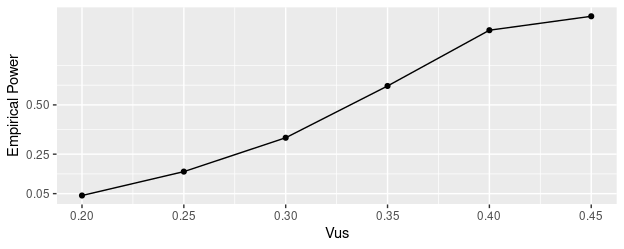For the simulation study, we additionally calculated the p-values of boot.test and varied $$VUS_1$$, $$VUS_2$$ and $$N$$.

# Additional functionality of the package

In this section we discuss functions of the package trinROC that help in the process of exploring, preparing and visualizing the data in the context of ROC analysis.

## How to apply the EDA function

Formal statistical testing is typically preceded by an exploratory data analysis. The function roc.eda serves this purpose and provides three different viewpoints on its input data:

• It computes the empirical or trinormal VUS as well as the test statistic for a single marker investigation
• It plots density, boxplots or scatter plots of the data according to the three classes
• It plots the empirical or trinormal ROC surface, using the package rgl.

The last option can be turned off by setting plotVUS = FALSE. If only the interactive three-dimensional plot is desired, one can also use the functions rocsurf.emp for empirical ROC surfaces and rocsurf.trin for trinormal ROC surfaces.

data( cancer)
roc.eda(dat = cancer[,c(1,5)], type = "trinormal", plotVUS = FALSE, saveVUS = TRUE)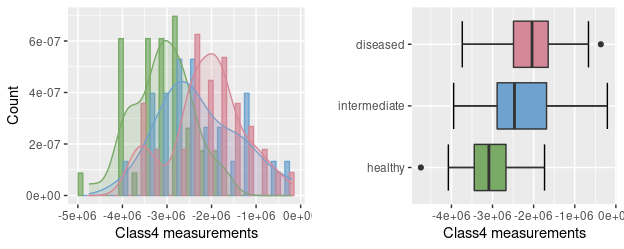#>
#>  Data overview of trinormal ROC Classifier
#> ---------------------------------------------------------------------
#>
#>  Applied tests: Trinormal based ROC and VUS test
#>  Significance level: 0.05
#>  Alternative hypothesis: two.sided
#> ---------------------------------------------------------------------
#>  data: healthy, intermediate and diseased
#>
#> ROC test statistic: 25.421, ROC p.value: 4e-05
#>  VUS test statistic:  3.418 ,  VUS p.value:  0.00063
#>
#>  trinormal VUS:  0.344
#>
#> Parameters:
#>      a   b   c   d
#>      1.3766  -1.1808 1.101   0.1951
#> ---------------------------------------------------------------------
roc.eda(dat = cancer[,c(1,5)], type = "empirical", sep.dens = TRUE, scatter = TRUE,
verbose = FALSE)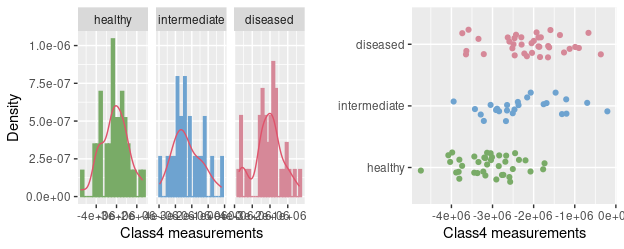## is last call is equal to:
# x <- with(cancer, cancer[trueClass=="healthy", 5])
# y <- with(cancer, cancer[trueClass=="intermediate", 5])
# z <- with(cancer, cancer[trueClass=="diseased", 5])
# roc.eda(x, y, z, type = "trinormal")

By setting plotVUS = TRUE an interactive rgl plot window is opened, displaying the ROC surface computed from the measurements. Depending the argument type the empirical or trinormal ROC surface is computed. The below Figures displays the empirical and trinormal snapshot of the ROC surfaces of the example data used in this section.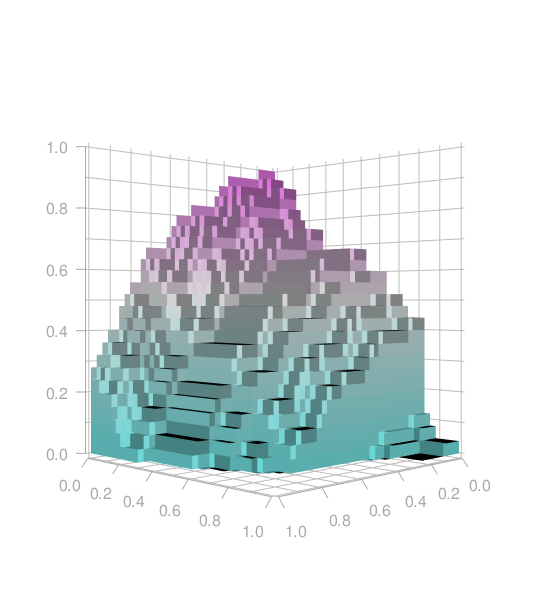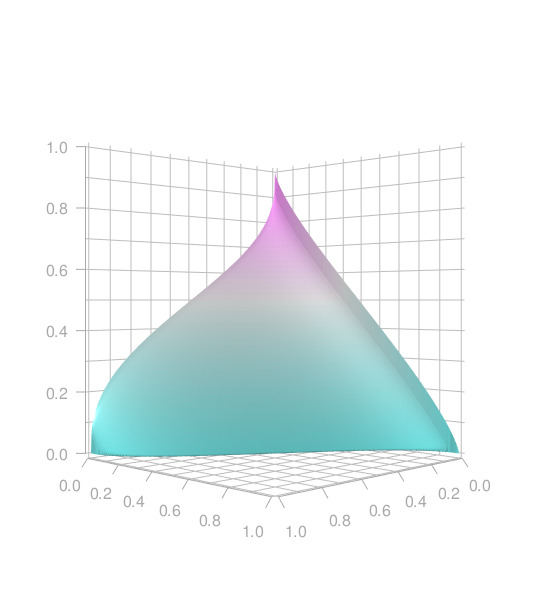Empirical and trinormal ROC surfaces

## Computing the (empirical) VUS

To calculate the VUS (empirical or estimate based on the trinormal assumption) the following code can be used.

data( cancer)
x <- with(cancer, cancer[trueClass=="healthy", 5])
y <- with(cancer, cancer[trueClass=="intermediate", 5])
z <- with(cancer, cancer[trueClass=="diseased", 5])
emp.vus(x, y, z)
#>  0.37741
trinVUS.test(x, y, z)$estimate #> VUS of Classifier 1 #> 0.34361 trinROC.test(x, y, z)$estimate
#>                  VUS
#> Classifier1: 0.34361

The ROC surface itself is visualized using rocsurf.emp(x, y, z) or rocsurf.trin(x, y, z).

## Transforming non-normal data using boxcoxROC

The trinormal model based test, trinROC.test or trinVUS.test are build upon a normality assumption of the data. If this assumption is violated, the trinormal tests may yield incorrect results. A common way to test for normality is the shapiro.test. If the hypothesis of normally distributed data is rejected, there is a possibility to apply the function boxcoxROC to the data. This function takes three vectors x, y and z and computes a Box-Cox transformation, see Box and Cox (1964) and Bantis et al. (2017). Consider this short example:

set.seed(712)
x <- rchisq(20, 2)
y <- rchisq(20, 6)
z <- rchisq(20, 10)
boxcoxROC(x, y, z)
#> ---------------------------------------------------------------------
#>  Optimal lambda       = 0.15
#>  Shift param. lambda2 = 0
#>
#>  Shapiro p-values for original data:
#>  x = 0.00079639, y = 0.0058895, z = 0.56616
#>
#>  Shapiro p-values for Box-Cox transformed data:
#>  x = 0.49498, y = 0.10501, z = 0.27073
#> ---------------------------------------------------------------------

## An omnibus analysis using the function roc3.test

The function roc3.test computes every one by one combination of classifiers it contains to a desired combination of the three statistical tests trinROC.test, trinVUS.test and boot.test in one step. Furthermore, single classifier assessment tests are automatically computed as well. The output consists of:

• Two data frames that contain information about each marker on its own. In the first data frame overview the markers are sorted by their empirical VUS, while in the second data frame they are shown in their original order.

• For each statistical test that has been chosen, two strictly upper triangular matrices are returned, one containing the p-values and one the test values.

out <- roc3.test(cancer[,1:8], type = c("ROC", "VUS"), paired = TRUE)
out[c(1,3)]
#> $Overview #> Class6 Class1 Class2 Class3 Class4 Class5 Class7 #> Charts 1.0000 2.0000 3.0000 4.0000 5.0000 6.0000 7.0000 #> Emp. VUS 0.5317 0.4763 0.4411 0.4354 0.3774 0.3661 0.2918 #> Trin. VUS 0.5557 0.4283 0.4158 0.4091 0.3436 0.3736 0.3440 #> p.value ROC test 0.0000 0.0000 0.0000 0.0000 0.0000 0.0000 0.0000 #> p.value VUS test 0.0000 0.0000 0.0000 0.0000 0.0006 0.0001 0.0007 #> Nr. of NA's 0.0000 0.0000 0.0000 0.0000 0.0000 0.0000 0.0000 #> #>$P.values
#> $P.values$trinROC
#>        Class1 Class2  Class3   Class4   Class5     Class6     Class7
#> Class1     NA 0.9708 0.91495 0.097375 0.873447 1.3541e-02 0.34697840
#> Class2     NA     NA 0.87303 0.034480 0.859044 1.5844e-02 0.46185397
#> Class3     NA     NA      NA 0.090533 0.101316 4.2548e-03 0.48086942
#> Class4     NA     NA      NA       NA 0.048306 4.6284e-05 0.11110658
#> Class5     NA     NA      NA       NA       NA 7.3777e-03 0.31660345
#> Class6     NA     NA      NA       NA       NA         NA 0.00090171
#> Class7     NA     NA      NA       NA       NA         NA         NA
#>
#> $P.values$trinVUS
#>        Class1  Class2  Class3  Class4  Class5    Class6    Class7
#> Class1     NA 0.80046 0.77512 0.15693 0.38661 0.1361267 0.2246178
#> Class2     NA      NA 0.91244 0.21143 0.43478 0.0886180 0.3066895
#> Class3     NA      NA      NA 0.28029 0.43850 0.0811900 0.4121852
#> Class4     NA      NA      NA      NA 0.60901 0.0094043 0.9957845
#> Class5     NA      NA      NA      NA      NA 0.0170064 0.6964812
#> Class6     NA      NA      NA      NA      NA        NA 0.0056033
#> Class7     NA      NA      NA      NA      NA        NA        NA

Several of the arguments of roc3.test are passed to the corresponding test functions specified by type (one or several of "ROC", "VUS", "Bootstrap"). These are (with corresponding default values) paired = FALSE, conf.level = 0.95 and n.boot = 1000.

The FDR p-value adjustment to be applied set p.adjust = TRUE.

roc3.test(cancer[,1:8], type = c("ROC", "VUS"), paired = TRUE,
p.adjust = TRUE)$P.values$trinROC
#>        Class1 Class2 Class3  Class4  Class5     Class6   Class7
#> Class1     NA 0.9708 0.9607 0.19342 0.96070 0.05545346 0.520468
#> Class2     NA     NA 0.9607 0.10344 0.96070 0.05545346 0.631141
#> Class3     NA     NA     NA 0.19342 0.19342 0.02978342 0.631141
#> Class4     NA     NA     NA      NA 0.12680 0.00097197 0.194437
#> Class5     NA     NA     NA      NA      NA 0.03873290 0.511436
#> Class6     NA     NA     NA      NA      NA         NA 0.009468
#> Class7     NA     NA     NA      NA      NA         NA       NA
out$P.values$trinROC
#>        Class1 Class2  Class3   Class4   Class5     Class6     Class7
#> Class1     NA 0.9708 0.91495 0.097375 0.873447 1.3541e-02 0.34697840
#> Class2     NA     NA 0.87303 0.034480 0.859044 1.5844e-02 0.46185397
#> Class3     NA     NA      NA 0.090533 0.101316 4.2548e-03 0.48086942
#> Class4     NA     NA      NA       NA 0.048306 4.6284e-05 0.11110658
#> Class5     NA     NA      NA       NA       NA 7.3777e-03 0.31660345
#> Class6     NA     NA      NA       NA       NA         NA 0.00090171
#> Class7     NA     NA      NA       NA       NA         NA         NA

## Some final Remarks

Xiong et al. (2007) also show how to apply the trinormal VUS test to a set of more than two classifiers at once.